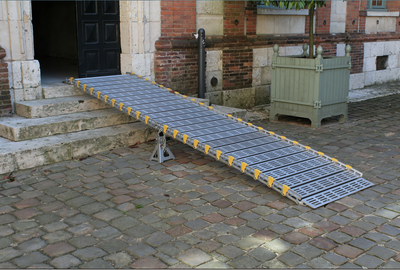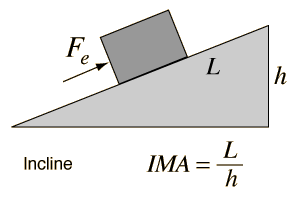# Inclined planeFigure 1: A ramp that is used to make it easier to lift an object up the stairs, using the idea of mechanical advantage.

Inclined planes, also referred to as ramps, are a type of simple machine which manipulate the direction and magnitude of a force. Inclined planes, like all other simple machines, use mechanical advantage which is the ratio of the output force to the applied force.

Ramps are used in many scenarios, and are used to make work against gravity easier (since the force decreases). Since the gravitational field acts perpendicular to the surface of the Earth, objects need energy in order to raise it a certain height. This is known as giving the object gravitational potential energy, which depends on the mass of the object, and how high it needs to go (and, oddly, what planet it's on).

Imagine trying to lift a boat out of the water onto land that is higher than the water. To lift it straight up would be a daunting task, so the use of ramps is common practice for such scenarios. The force required to lift the boat to land is considerably less, but the distance needed to pull the boat is much longer.Figure 2: The mechanical advantage of an inclined plane, equal to the length of the plane divided by the height.

The mechanical advantage for a ramp is the ratio of the force applied to the output force. By conservation of energy, the mechanical advantage can be expressed in terms of the distance traveled. Seen in Figure 2, the ideal mechanical advantage for an inclined plane is simply where L is the length of the plane, and h is the height.

This means that the longer the plane gets, the easier the task will be in terms of force, but the distance will always have to change in proportion. This is because as the length gets very long, the angle of elevation of the ramp decreases. As increases the ramp has such a low elevation that it is essentially like walking on a flat plane.

Remember that this is only for an ideal ramp, where there are no non-conservative forces acting on the object. There is never a time in reality when friction can be ignored fully, so the true mechanical advantage will always be less than the ideal mechanical advantage. While ramps are used all the time (even stairs are a effectively a form of ramp), friction on the ramp creates situations where there's an upper limit to how helpful they are in saving effort.